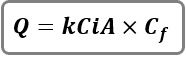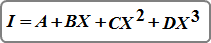# The Rational Method

The Rational method was developed over 100 years ago and continues to be used for urban watershed modeling, typically on areas less than about 20 acres but is valid up to 200 acres. While there exists many varieties of the Rational method, Stormwater Studio uses the most popular… the Standard Rational.

## Standard Rational

The Standard Rational method computes the peak discharge as determined by the well known Rational formula.Where:

Qp = peak discharge, cfs (cms)
k = 1 English (0.00278 Metric)
C = runoff coefficient
A = basin area, acres (hectares)
i = intensity, in/hr (mm/hr)
Cf = frequency correction factor where C x Cf <= 1.0

The rainfall intensity is computed by one of the following equations:

### FHA EquationWhere:

B, D and E are constants
Tc = time of concentration in minutes subject to the Minimum Tc

### Third Degree Polynomial EquationWhere:

I = rainfall intensity in in/hr (mm/hr)
X = Ln(time duration in minutes)
A = coefficient
B = coefficient
C = coefficient
D = coefficient

Appropriate values for X are 8 to 180 minutes subject to the Minimum Tc.
The constants are pre-computed by the program, or as manually entered, and are based on geographic location.